Showing 131 to 140 of 338 results.
0%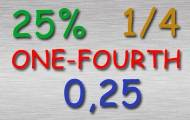## Convert fractions, percentages and decimals

• Fractions
• Percents
• Decimals
• Conversion
Practice convert a number between fraction, percentage and decimal. Includes the ten steps from a whole of one-tenth.
0%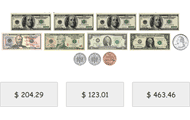## Count money

• Money
• Counting
• Mental calculation
Look at the notes and coins and add up the sum. Practicing basic knowledge in how to count money with increasing difficulty. This knowledge game uses all valid denominations of the Swedish krona, U.S.
0%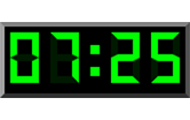## Digital clock in English

• Clock
• Digital clock
• Numbers
Practice the digital clock with 24 hours. Read a written time and find the corresponding time among the three digital clocks.
0%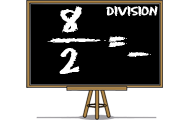## Division

• Numbers
• Division
• Mental calculation
Practice division, ie dividing two numbers. Find the quotients of the numerators divided by the denominators in this math game.
0%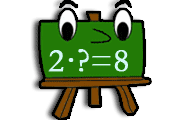## Find the factor

• Factor
• Multiplication
• Product
Try to find the requested factors in this game of multiplication. You get a factor and a product and your task is to find the missing product.
0%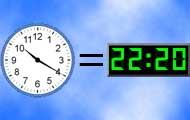## Match clocks

• Clock
• Analog clock
• Digital clock
• Numbers
Match clocks that can be both analog and digital. Practice telling time with both types of clocks.
0%## Minecraft math

• Multiplication
• Division
• Subtraction
• Problem solving
Count with Minecraft! You will need to multiply, divide and subtract.
0%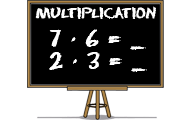## Multiplication

• Numbers
• Multiplication
• Mental calculation
Learn multiplication with this math game for students. Find the product of the factors in all times tables.
0%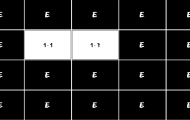## Multiplication memory

• Multiplication
• Times tables
• Mental arithmetic
• Memory training
Practice multiplication with times tables in this memory game where you should match an expression with the right product.
0%## Number bonds

• Numbers
• Mental calculation
• Number bonds
Try to find the number that is a number bond to another number and adds up to the given sum. Practice part-part-whole relationships to the numbers 0-20 and facilitates the important mental calculation
Weekly top list
1.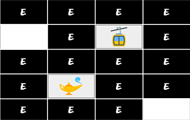Brain training
2.Brain training
3.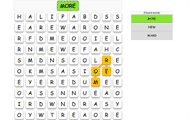English
4.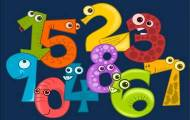Spanish
5.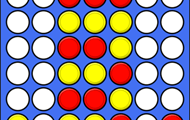Brain training

More plus membership benefits